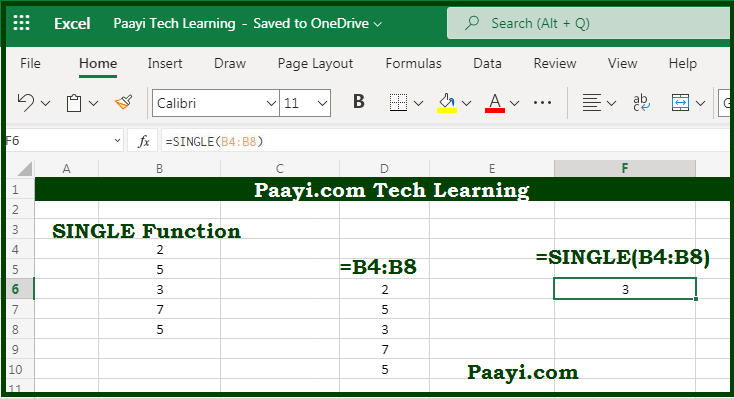# Learn How to Use Microsoft Excel SINGLE Function

Written by | 0 Comments | 1005 Views

In this article, you will learn how to use the Microsoft Excel SINGLE function and its prime function in Microsoft Excel. You will also get to know the Microsoft Excel SINGLE function return value and syntax with the help of some examples.

Microsoft Excel SINGLE Function

The primary function of the Microsoft Excel SINGLE function is to get a single value with the implicit intersection. That implies that you can return a single value using the implicit intersection with the help of the SINGLE function. The Microsoft Excel SINGLE function was introduced with the "Dynamic Arrays" but further deprecated in favor of @ character.

Return Value of SINGLE Function

The return value will be the cell or value at the implied intersection.

Syntax of SINGLE Function

=SINGLE(value)

Where the arguments:

value: This is the value to evaluate using the implicit intersection.

## How to Use Microsoft Excel SINGLE Function?As we know, the Microsoft Excel SINGLE function is to get a single value with the implicit intersection. The SINGLE function is basically for the backward compatibility with implicit intersection formulas in previous versions of Microsoft Excel.

When the argument supplied is a range, the SINGLE function will return the cell at the intersection of the cell formula's row or column. If there is no intersection or more than one intersection, SINGLE returns a #VALUE! Error.